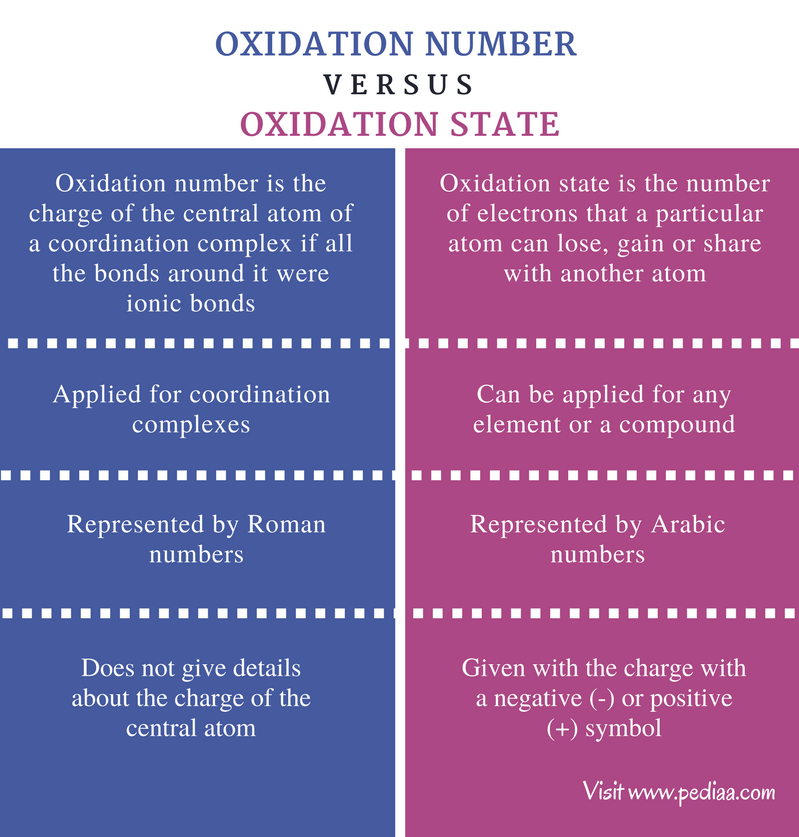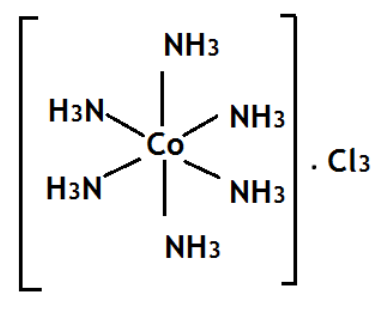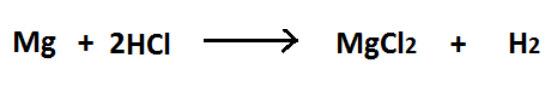# Difference Between Oxidation Number and Oxidation State

## Main Difference – Oxidation Number vs Oxidation State

Oxidation number and oxidation state are often considered as the same. This is because the oxidation state can be equal to the oxidation number of a particular atom according to the type of compound it is located in. However, there is a slight difference between oxidation number and oxidation state. The main difference between oxidation number and oxidation state is that oxidation number is the charge of the central atom of a coordination complex if all the bonds around it were ionic bonds whereas oxidation state is the number of electrons that a particular atom can lose, gain or share with another atom.

### Key Areas Covered

1. What is Oxidation Number
– Definition, Rules, Examples
2. What is Oxidation State
– Definition, Rules, Examples
3. What is the Difference Between Oxidation Number and Oxidation State
– Comparison of Key Differences

Key Terms: Arabic Numbers, Bidentate, Coordination Bonds, Coordination Complex, Coordination Number, Ionic Bonds, Ligands, Monodentate, Oxidation Number, Oxidation State, Roman Numbers## What is Oxidation Number

The oxidation number can be defined as the charge of the central atom of a coordination complex if all the bonds around it were ionic bonds. A coordination complex is a chemical structure composed of a central metal atom that is bonded to several ligands. These are also called metal complexes due to the presence of a central metal atom. This central metal atom is called the coordination center. Ligands are chemical groups that are attached to the metal atom through coordination bonds (coordination covalent bonds). A coordination compound contains several coordination complexes.

Most of the times, the central metal atom is a transition metal (d-block element). The coordination bond is formed when an electron rich species donate its electron pairs (one or two pairs) to the central atom. These donors are called ligands. A ligand can either be a neutral molecule or a negative charged ion. This coordination bond is a covalent bond because it is formed due to electron sharing between two atoms. The number of donor atoms bonded to the central atom is called the coordination number.

There can be either monodentate or bidentate ligands. A monodentate ligand binds with the central atom through a single coordination bond whereas a bidentate ligand binds through two coordination bond. The coordination number decides the geometry of a coordination complex. However, coordination number is not the oxidation number of the central atom. But sometimes, the coordination number may equal the oxidation number.Figure 01: A coordination complex of cobalt

The above image shows the [Co (NH3)6] Cl3 complex. The coordination number of Cobalt is 6 because there are six ligands of ammonia bonded to cobalt atom. But the oxidation number of cobalt is three because the oxidation number is the charge of the central atom of a coordination complex if all the ligands around it are removed. As the three Cl atoms cause -3 charge, and ammonia molecules are neutrally charged, the cobalt atom should be +3 charged in order to neutralize the charge coming from Cl atoms. Therefore, the oxidation number of Cobalt is written as Co (III).

## What is Oxidation State

The oxidation state can be defined as the number of electrons that a particular atom can lose, gain or share with another atom. This term is not only limited to coordination complexes. Oxidation state actually gives the degree of oxidation of an atom in a compound. The oxidation state is always given as a whole number, and it is represented in Hindu-Arabic numbers, including the charge of the atom.

### Rules for Determining Oxidation State

The oxidation state of an atom is given under seven rules.

#### Rule 1

A single element and the compounds composed of a single element have zero oxidation state per each atom.

For example, the oxidation state of Nitrogen (N) in the compound N2 is zero.

#### Rule 2

The total charge of a compound is the sum of the charges of each element.

For example, in a neutral species such as NaCl, the total charge is zero. Therefore, the oxidation state of each element should be given as Na (+1) and Cl (-1).

In ionic species, such as NH4+, the sum of the oxidation states of N and H atoms should be equal to the overall charge.

(N + 4H) = (-3) + (1 x 4) = +1

#### Rule 3

The oxidation state for group 1 metals (1A) is always +1, and for group 2 metals (2A), it is always +2.

For example, the oxidation state of Lithium (Li) would always be +1, and the oxidation state of Magnesium (Mg) would always be +2.

#### Rule 4

The oxidation state of Fluorine (F) is always -1.

Remember the oxidation state of Fluorine in F2 is zero according to the first rule.

#### Rule 5

The most electronegative atom compared to other atoms bonded to it, is given the negative charge.

For example, Fluorine is more electronegative than Hydrogen. Therefore, in HF, the oxidation state of hydrogen is -1. But typically the oxidation state of hydrogen is +1.

#### Rule 6

The oxygen state of Hydrogen (H) is normally +1.

Hydrogen atom easily loses an electron, forming its cation. But when Hydrogen is in combination with a group 1A or a 2A metal, then the oxidation state of Hydrogen is -1. For example, NaH.

#### Rule 7

The oxidation state of oxygen is normally -2.

Oxygen is more electronegative and attracts electrons. Therefore, it easily forms -2 oxidation state. But in peroxides, the oxidation number is -1 since two oxygen atoms are bonded in single bond there.

The oxidation state is very useful for the determination of products in redox reactions. Redox reactions are chemical reactions that include electron exchange between atoms. In redox reactions, two half reactions occur parallel. One is the oxidation reaction, and the other one is the reduction reaction. The oxidation reaction involves the increase of oxidation state of an atom while reduction reaction involves the decrease of the oxidation of an atom.Figure 02: Redox Reaction between Mg and H2

In the above reaction, Mg is a single element having zero (0) oxidation state. But after the reaction with H2, it has formed MgCl2, and the oxidation state of Mg is +2. The oxidation state of Mg is increased here. Therefore, it is the oxidation half reaction in this redox reaction. The oxidation state of H in HCl is +1. But the product H2 is in zero (0) oxidation state. The oxidation state has been decreased. Therefore, it is the reduction half reaction in this redox reaction.

## Difference Between Oxidation Number and Oxidation State

### Definition

Oxidation Number: The oxidation number can be defined as the charge of the central atom of a coordination complex if all the bonds around it were ionic bonds.

Oxidation State: The oxidation state can be defined the number of electrons that a particular atom can lose, gain or share with another atom.

### Application

Oxidation Number: Oxidation number is applied for coordination complexes.

Oxidation State: Oxidation state can be applied for any element or a compound.

### Representation of Number

Oxidation Number: Roman numbers are used to represent the oxidation number.

Oxidation State: Hindu-Arabic numbers are used to represent the oxidation state.

### Representation of the Charge

Oxidation Number: The oxidation number does not give details about the charge of the central atom.

Oxidation State: The oxidation state is given with the charge with a negative (-) or positive (+) symbol.

### Conclusion

For a normal element or a compound (instead of coordination complexes), the oxidation state and the oxidation number are the same. But when considering all the compounds including coordination compounds, there is a slight difference. The main difference between oxidation number and oxidation state is that oxidation number is the charge of the central atom of a coordination complex if all the bonds around it were ionic bonds whereas oxidation state is the number of electrons that a particular atom can lose, gain or share with another atom.

##### References:

1.“Oxidation states (oxidation numbers)”. Oxidation states (oxidation numbers) N.p., n.d. Web. Available here. 05 July 2017.
2.”Rules for Assigning Oxidation Numbers to Elements.” Dummies. N.p., n.d. Web. Available here. 05 July 2017.
3.”Coordination Compounds.” Coordination Compounds Help Page. N.p., n.d. Web. Available here. 05 July 2017.